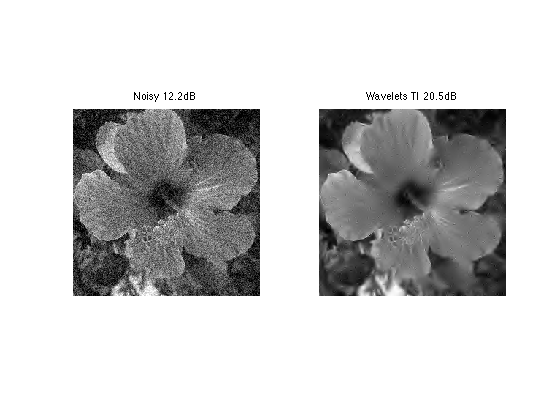$\newcommand{\NN}{\mathbb{N}} \newcommand{\CC}{\mathbb{C}} \newcommand{\GG}{\mathbb{G}} \newcommand{\LL}{\mathbb{L}} \newcommand{\PP}{\mathbb{P}} \newcommand{\QQ}{\mathbb{Q}} \newcommand{\RR}{\mathbb{R}} \newcommand{\VV}{\mathbb{V}} \newcommand{\ZZ}{\mathbb{Z}} \newcommand{\FF}{\mathbb{F}} \newcommand{\KK}{\mathbb{K}} \newcommand{\UU}{\mathbb{U}} \newcommand{\EE}{\mathbb{E}} \newcommand{\Aa}{\mathcal{A}} \newcommand{\Bb}{\mathcal{B}} \newcommand{\Cc}{\mathcal{C}} \newcommand{\Dd}{\mathcal{D}} \newcommand{\Ee}{\mathcal{E}} \newcommand{\Ff}{\mathcal{F}} \newcommand{\Gg}{\mathcal{G}} \newcommand{\Hh}{\mathcal{H}} \newcommand{\Ii}{\mathcal{I}} \newcommand{\Jj}{\mathcal{J}} \newcommand{\Kk}{\mathcal{K}} \newcommand{\Ll}{\mathcal{L}} \newcommand{\Mm}{\mathcal{M}} \newcommand{\Nn}{\mathcal{N}} \newcommand{\Oo}{\mathcal{O}} \newcommand{\Pp}{\mathcal{P}} \newcommand{\Qq}{\mathcal{Q}} \newcommand{\Rr}{\mathcal{R}} \newcommand{\Ss}{\mathcal{S}} \newcommand{\Tt}{\mathcal{T}} \newcommand{\Uu}{\mathcal{U}} \newcommand{\Vv}{\mathcal{V}} \newcommand{\Ww}{\mathcal{W}} \newcommand{\Xx}{\mathcal{X}} \newcommand{\Yy}{\mathcal{Y}} \newcommand{\Zz}{\mathcal{Z}} \newcommand{\al}{\alpha} \newcommand{\la}{\lambda} \newcommand{\ga}{\gamma} \newcommand{\Ga}{\Gamma} \newcommand{\La}{\Lambda} \newcommand{\Si}{\Sigma} \newcommand{\si}{\sigma} \newcommand{\be}{\beta} \newcommand{\de}{\delta} \newcommand{\De}{\Delta} \renewcommand{\phi}{\varphi} \renewcommand{\th}{\theta} \newcommand{\om}{\omega} \newcommand{\Om}{\Omega} \renewcommand{\epsilon}{\varepsilon} \newcommand{\Calpha}{\mathrm{C}^\al} \newcommand{\Cbeta}{\mathrm{C}^\be} \newcommand{\Cal}{\text{C}^\al} \newcommand{\Cdeux}{\text{C}^{2}} \newcommand{\Cun}{\text{C}^{1}} \newcommand{\Calt}{\text{C}^{#1}} \newcommand{\lun}{\ell^1} \newcommand{\ldeux}{\ell^2} \newcommand{\linf}{\ell^\infty} \newcommand{\ldeuxj}{{\ldeux_j}} \newcommand{\Lun}{\text{\upshape L}^1} \newcommand{\Ldeux}{\text{\upshape L}^2} \newcommand{\Lp}{\text{\upshape L}^p} \newcommand{\Lq}{\text{\upshape L}^q} \newcommand{\Linf}{\text{\upshape L}^\infty} \newcommand{\lzero}{\ell^0} \newcommand{\lp}{\ell^p} \renewcommand{\d}{\ins{d}} \newcommand{\Grad}{\text{Grad}} \newcommand{\grad}{\text{grad}} \renewcommand{\div}{\text{div}} \newcommand{\diag}{\text{diag}} \newcommand{\pd}{ \frac{ \partial #1}{\partial #2} } \newcommand{\pdd}{ \frac{ \partial^2 #1}{\partial #2^2} } \newcommand{\dotp}{\langle #1,\,#2\rangle} \newcommand{\norm}{|\!| #1 |\!|} \newcommand{\normi}{\norm{#1}_{\infty}} \newcommand{\normu}{\norm{#1}_{1}} \newcommand{\normz}{\norm{#1}_{0}} \newcommand{\abs}{\vert #1 \vert} \newcommand{\argmin}{\text{argmin}} \newcommand{\argmax}{\text{argmax}} \newcommand{\uargmin}{\underset{#1}{\argmin}\;} \newcommand{\uargmax}{\underset{#1}{\argmax}\;} \newcommand{\umin}{\underset{#1}{\min}\;} \newcommand{\umax}{\underset{#1}{\max}\;} \newcommand{\pa}{\left( #1 \right)} \newcommand{\choice}{ \left\{ \begin{array}{l} #1 \end{array} \right. } \newcommand{\enscond}{ \left\{ #1 \;:\; #2 \right\} } \newcommand{\qandq}{ \quad \text{and} \quad } \newcommand{\qqandqq}{ \qquad \text{and} \qquad } \newcommand{\qifq}{ \quad \text{if} \quad } \newcommand{\qqifqq}{ \qquad \text{if} \qquad } \newcommand{\qwhereq}{ \quad \text{where} \quad } \newcommand{\qqwhereqq}{ \qquad \text{where} \qquad } \newcommand{\qwithq}{ \quad \text{with} \quad } \newcommand{\qqwithqq}{ \qquad \text{with} \qquad } \newcommand{\qforq}{ \quad \text{for} \quad } \newcommand{\qqforqq}{ \qquad \text{for} \qquad } \newcommand{\qqsinceqq}{ \qquad \text{since} \qquad } \newcommand{\qsinceq}{ \quad \text{since} \quad } \newcommand{\qarrq}{\quad\Longrightarrow\quad} \newcommand{\qqarrqq}{\quad\Longrightarrow\quad} \newcommand{\qiffq}{\quad\Longleftrightarrow\quad} \newcommand{\qqiffqq}{\qquad\Longleftrightarrow\qquad} \newcommand{\qsubjq}{ \quad \text{subject to} \quad } \newcommand{\qqsubjqq}{ \qquad \text{subject to} \qquad }$

Denoising by Sobolev and Total Variation Regularization

# Denoising by Sobolev and Total Variation Regularization

This numerical tour explores the use of variational minimization to perform denoising. It consider the Sobolev and the Total Variation regularization functional (priors).

## Installing toolboxes and setting up the path.

You need to download the following files: signal toolbox and general toolbox.

You need to unzip these toolboxes in your working directory, so that you have toolbox_signal and toolbox_general in your directory.

For Scilab user: you must replace the Matlab comment '%' by its Scilab counterpart '//'.

Recommandation: You should create a text file named for instance numericaltour.sce (in Scilab) or numericaltour.m (in Matlab) to write all the Scilab/Matlab command you want to execute. Then, simply run exec('numericaltour.sce'); (in Scilab) or numericaltour; (in Matlab) to run the commands.

Execute this line only if you are using Matlab.

getd = @(p)path(p,path); % scilab users must *not* execute this


Then you can add the toolboxes to the path.

getd('toolbox_signal/');
getd('toolbox_general/');


## Prior and Regularization

For a given image $$f$$, a prior $$J(f) \in \mathbb{R}$$ assign a score supposed to be small for the image of interest.

A denoising of some noisy image $$y$$ is obtained by a variational minimization that mixes a fit to the data (usually using an $$L^2$$ norm) and the prior. $\min_f \frac{1}{2}\|y-f\|^2 + \lambda J(f)$

If $$J(f)$$ is a convex function of $$f$$, then the minimum exists and is unique.

The parameter $$\tau>0$$ should be adapted to the noise level. Increasing its value means a more agressive denoising.

If $$J(f)$$ is a smooth functional of the image $$f$$, a minimizer of this problem can be computed by gradient descent. It defines a series of images $$f^{(\ell)}$$ indexed by $$\ell \in \mathbb{N}$$ as $f^{(\ell+1)} = f^{(\ell)} + \tau \left( f^{(\ell)}-y + \lambda \text{Grad}J(f^{(\ell)}) \right).$

Note that for $$f^{(\ell)}$$ to converge with $$\ell \rightarrow +\infty$$ toward a solution of the problem, $$\tau$$ needs to be small enough, more precisely, if the functional $$J$$ is twice differentiable, $\tau < \frac{2}{1 + \lambda \max_f \|D^2 J(f)\|}.$

## Sobolev Prior

The Sobolev image prior is a quadratic prior, i.e. an Hilbert (pseudo)-norm.

First we load a clean image.

n = 256;
name = 'hibiscus';
f0 = load_image(name,n);
f0 = rescale( sum(f0,3) );


For a smooth continuous function $$f$$ it is defined as $J(f) = \int \|\nabla f(x)\|^2 d x$

Where the gradient vector at point $$x$$ is defined as $\nabla f(x) = \left( \frac{\partial f(x)}{\partial x_1},\frac{\partial f(x)}{\partial x_2} \right)$

For a discrete pixelized image $$f \in \mathbb{R}^N$$ where $$N=n \times n$$ is the number of pixel, $$\nabla f(x) \in \mathbb{R}^2$$ is computed using finite difference.

Gr = grad(f0);


One can compute the norm of gradient, $$d(x) = \|\nabla f(x)\|$$.

d = sqrt(sum3(Gr.^2,3));


Display.

clf;
imageplot(Gr, strcat(['grad']), 1,2,1);
imageplot(d, strcat(['|grad|']), 1,2,2);The Sobolev norm is the (squared) $$L^2$$ norm of $$\nabla f \in \mathbb{R}^{N \times 2}$$.

sob = sum(d(:).^2);


## Heat Regularization for Denoising

Heat regularization smoothes the image using a low pass filter. Increasing the value of \lambda increases the amount of smoothing.

Add some noise to the original image.

sigma = .1;
y = f0 + randn(n,n)*sigma;


The solution $$f^{\star}$$ of the Sobolev regularization can be computed exactly using the Fourier transform. $\hat f^{\star}(\omega) = \frac{\hat y(\omega)}{ 1+\lambda S(\omega) } \quad\text{where}\quad S(\omega)=\|\omega\|^2.$

This shows that $$f^{\star}$$ is a filtering of $$y$$.

Useful for later: Fourier transform of the observations.

yF = fft2(y);


Compute the Sobolev prior penalty S (rescale to [0,1]).

x = [0:n/2-1, -n/2:-1];
[Y,X] = meshgrid(x,x);
S = (X.^2 + Y.^2)*(2/n)^2;


Regularization parameter:

lambda = 20;


Perform the denoising by filtering.

fSob = real( ifft2( yF ./ ( 1 + lambda*S) ) );


Display.

clf;
imageplot(clamp(fSob));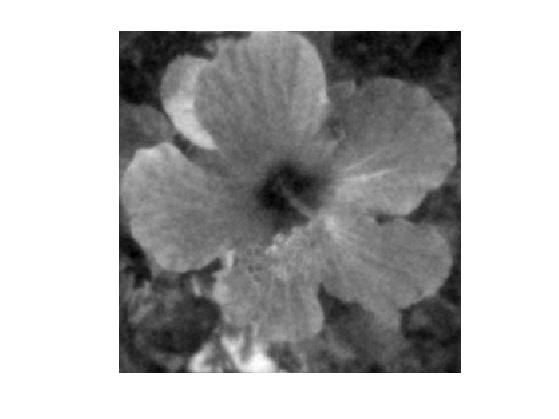Exercice 1: (check the solution) Compute the solution for several value of $$\lambda$$ and choose the optimal lambda and the corresponding optimal denoising fSob0. You can increase progressively lambda and reduce considerably the number of iterations.

exo1;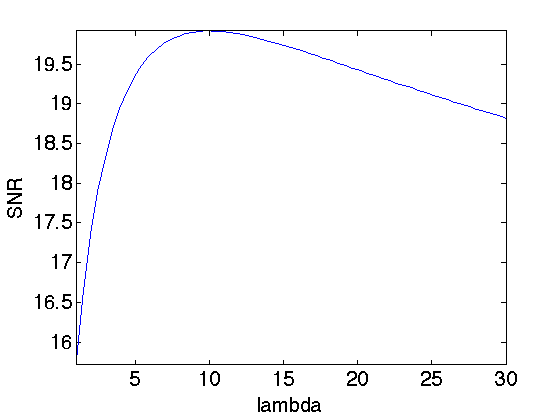Display best "oracle" denoising result.

esob = snr(f0,fSob0);  enoisy = snr(f0,y);
clf;
imageplot(clamp(y), strcat(['Noisy ' num2str(enoisy,3) 'dB']), 1,2,1);
imageplot(clamp(fSob0), strcat(['Sobolev regularization ' num2str(esob,3) 'dB']), 1,2,2);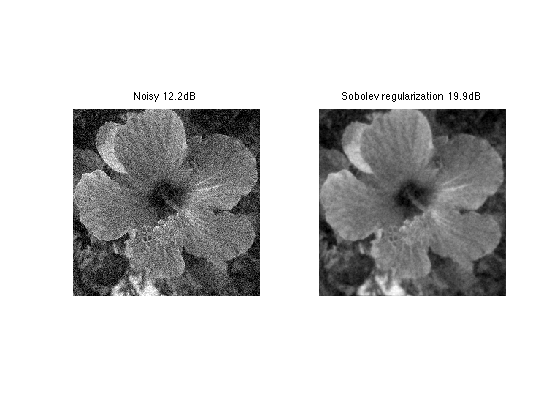## Total Variation Prior

The total variation is a Banach norm. On the contrary to the Sobolev norm, it is able to take into account step edges.

The total variation of a smooth image $$f$$ is defined as $J(f)=\int \|\nabla f(x)\| d x$

It is extended to non-smooth images having step discontinuities.

The total variation of an image is also equal to the total length of its level sets. $J(f)=\int_{-\infty}^{+\infty} L( S_t(f) ) dt$

Where $$S_t(f)$$ is the level set at $$t$$ of the image $$f$$ $S_t(f)=\{ x \backslash f(x)=t \}$

Exercice 2: (check the solution) Compute the total variation of f0.

exo2;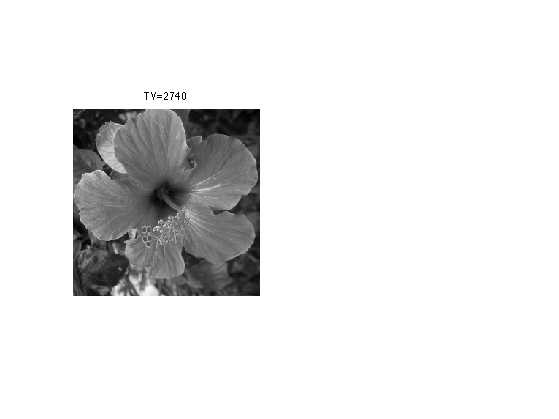The Gradient of the TV norm is $\text{Grad}J(f) = \text{div}\left( \frac{\nabla f}{\|\nabla f\|} \right) .$

The gradient of the TV norm is not defined if at a pixel $$x$$ one has $$\nabla f(x)=0$$. This means that the TV norm is difficult to minimize, and its gradient flow is not well defined.

To define a gradient flow, we consider instead a smooth TV norm $J_\epsilon(f) = \int \sqrt{ \varepsilon^2 + \| \nabla f(x) \|^2 } d x$

This corresponds to replacing $$\|u\|$$ by $$\sqrt{\varepsilon^2 + \|u\|^2}$$ which is a smooth function.

We display (in 1D) the smoothing of the absolute value.

u = linspace(-5,5)';
clf;
subplot(2,1,1); hold('on');
plot(u, abs(u), 'b');
plot(u, sqrt(.5^2+u.^2), 'r');
title('\epsilon=1/2'); axis('square');
subplot(2,1,2); hold('on');
plot(u, abs(u), 'b');
plot(u, sqrt(1^2+u.^2), 'r');
title('\epsilon=1'); axis('square');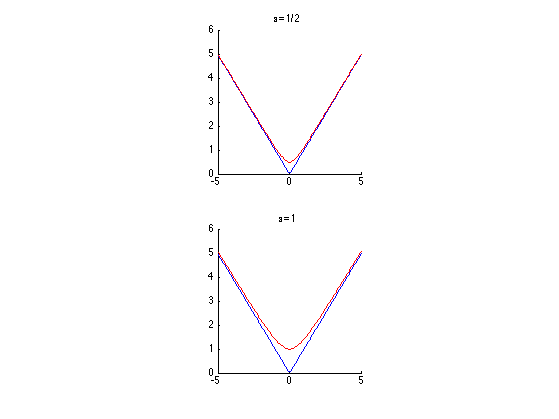The Gradient of the smoothed TV norm is $\text{Grad}J(f) = \text{div}\left( \frac{\nabla f}{\sqrt{\varepsilon^2 + \|\nabla f\|^2}} \right) .$

When $$\varepsilon$$ increases, the smoothed TV gradient approaches the Laplacian (normalized by $$1/\varepsilon$$).

epsilon_list = [1e-9 1e-2 1e-1 .5];
clf;
for i=1:length(epsilon_list)
G = div( Gr ./ repmat( sqrt( epsilon_list(i)^2 + d.^2 ) , [1 1 2]) );
imageplot(G, strcat(['epsilon=' num2str(epsilon_list(i))]), 2,2,i);
end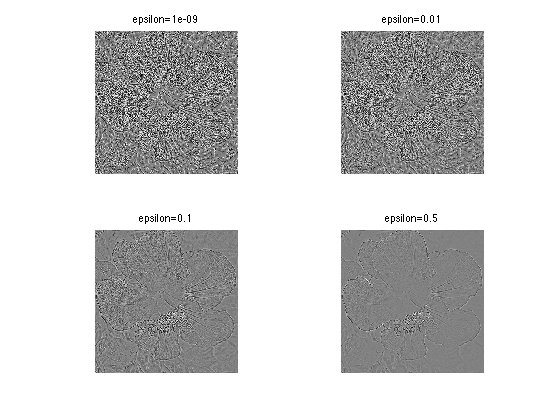## Total Variation Regulariation for Denoising

Total variation regularization was introduced by Rudin, Osher and Fatemi, to better respect the edge of image than linear filtering.

We set a small enough regularization parameter.

epsilon = 1e-2;


Define the regularization parameter $$\lambda$$.

lambda = .1;


The step size for diffusion should satisfy: $\tau < \frac{2}{1 + \lambda 8 / \varepsilon} .$

tau = 2 / ( 1 + lambda * 8 / epsilon);


Initialization of the minimization.

fTV = y;


Compute the gradient of the smoothed TV norm.

Gr = grad(fTV);
d = sqrt(sum3(Gr.^2,3));
G = -div( Gr ./ repmat( sqrt( epsilon^2 + d.^2 ) , [1 1 2]) );


One step of descent.

fTV = fTV - tau*( y-fTV + lambda* G);


Exercice 3: (check the solution) Compute the gradient descent and monitor the minimized energy.

exo3;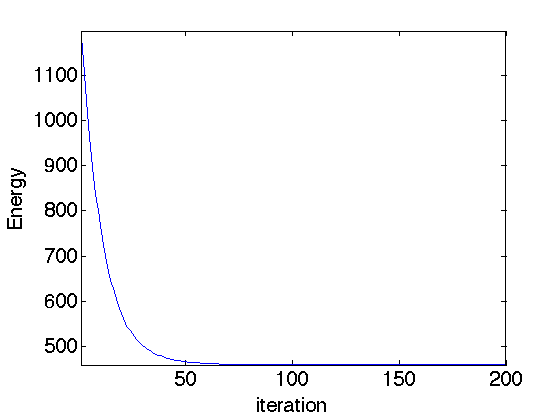Display the image.

clf;
imageplot(fTV);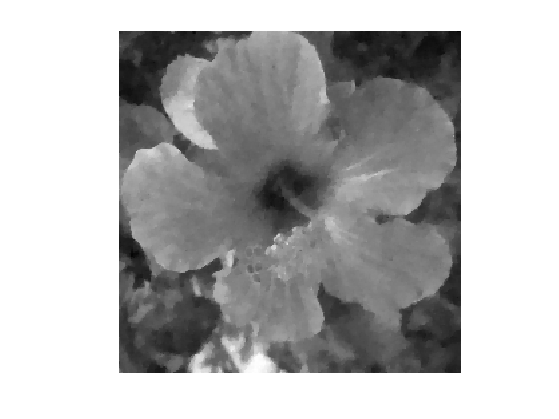Exercice 4: (check the solution) Compute the solution for several value of $$\lambda$$ and choose the optimal $$\lambda$$ and the corresponding optimal denoising fSob0. You can increase progressively $$\lambda$$ and reduce considerably the number of iterations.

exo4;Display best "oracle" denoising result.

etvr = snr(f0,fTV0);
clf;
imageplot(clamp(y), strcat(['Noisy ' num2str(enoisy,3) 'dB']), 1,2,1);
imageplot(clamp(fTV0), strcat(['TV regularization ' num2str(etvr,3) 'dB']), 1,2,2);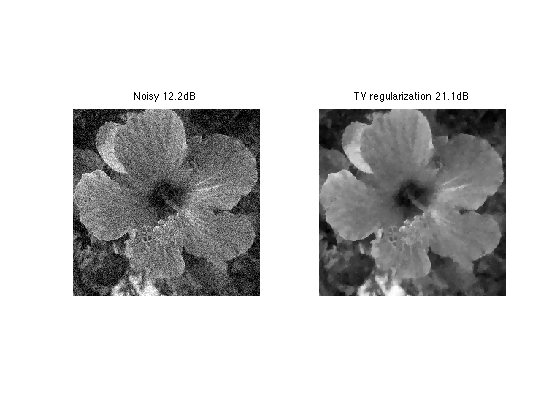Exercice 5: (check the solution) Compare the TV denoising with a hard thresholding in a translation invariant tight frame of wavelets.

exo5;